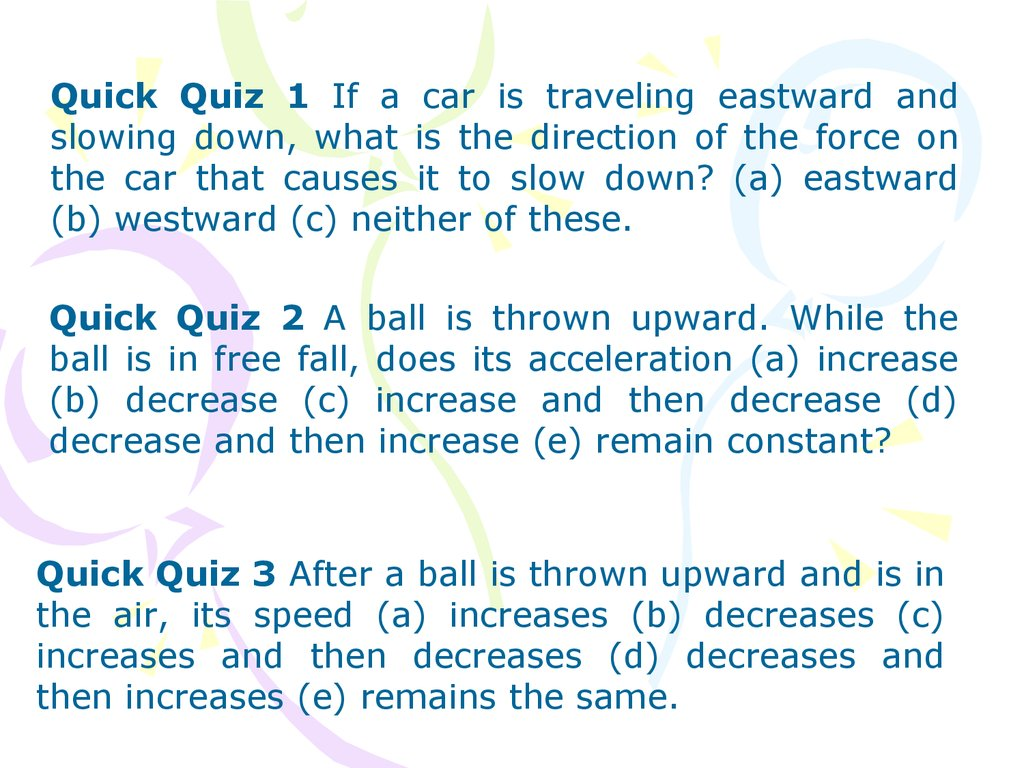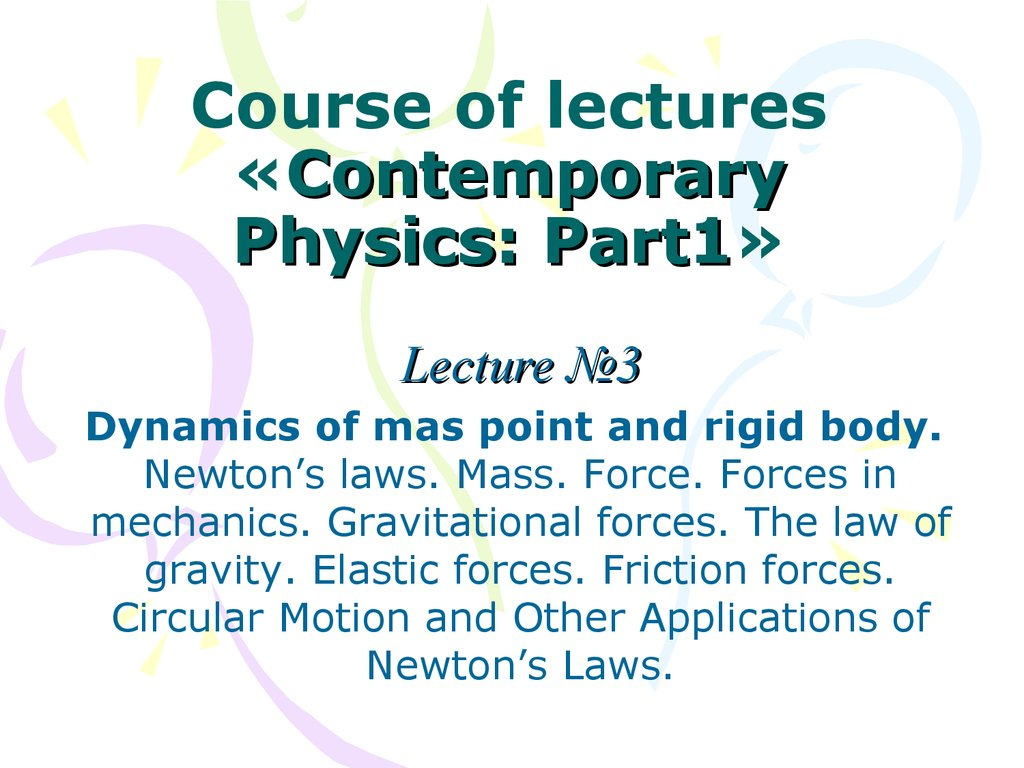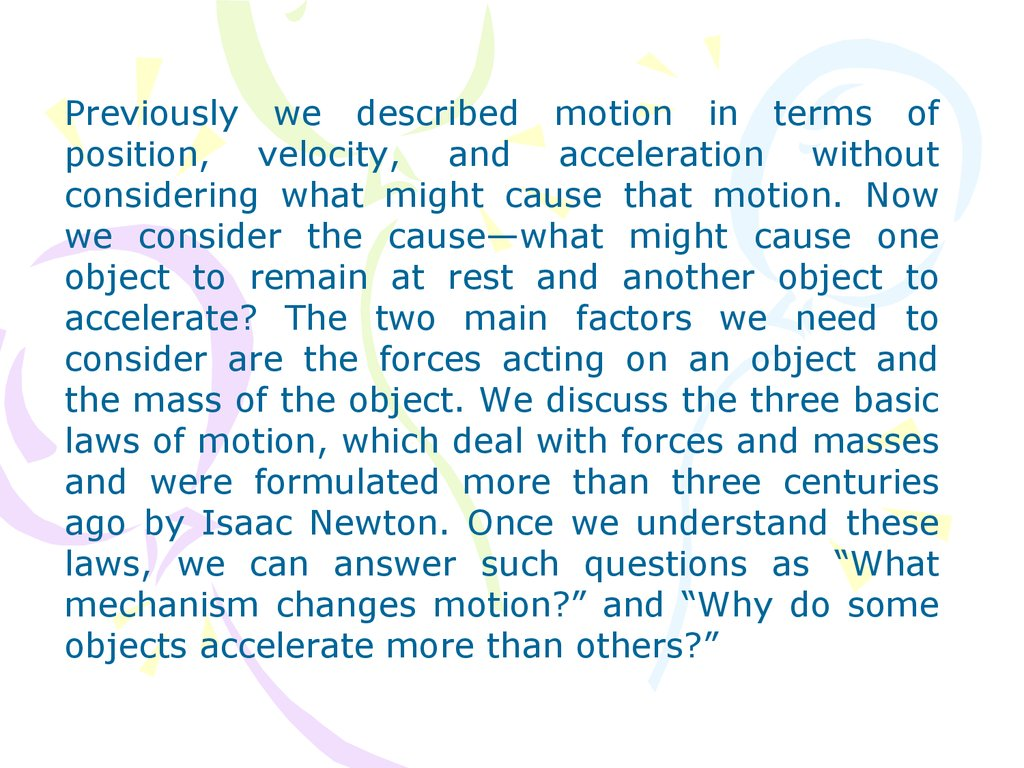# Quick Quiz

## 1.

Quick Quiz 1 If a car is traveling eastward and
slowing down, what is the direction of the force on
the car that causes it to slow down? (a) eastward
(b) westward (c) neither of these.
Quick Quiz 2 A ball is thrown upward. While the
ball is in free fall, does its acceleration (a) increase
(b) decrease (c) increase and then decrease (d)
decrease and then increase (e) remain constant?
Quick Quiz 3 After a ball is thrown upward and is in
the air, its speed (a) increases (b) decreases (c)
increases and then decreases (d) decreases and
then increases (e) remains the same.

## 2. Course of lectures «Contemporary Physics: Part1»

Part1
Lecture №3
Dynamics of mas point and rigid body.
Newton’s laws. Mass. Force. Forces in
mechanics. Gravitational forces. The law of
gravity. Elastic forces. Friction forces.
Circular Motion and Other Applications of
Newton’s Laws.

## 3.

Previously we described motion in terms of
position, velocity, and acceleration without
considering what might cause that motion. Now
we consider the cause—what might cause one
object to remain at rest and another object to
accelerate? The two main factors we need to
consider are the forces acting on an object and
the mass of the object. We discuss the three basic
laws of motion, which deal with forces and masses
and were formulated more than three centuries
ago by Isaac Newton. Once we understand these
laws, we can answer such questions as “What
mechanism changes motion?” and “Why do some
objects accelerate more than others?”

## 4.

field forces
contact forces
The Concept of Force

## 5.

The Concept of Force
The only known fundamental forces in nature are
all field forces:
(1) gravitational forces between objects,
(2) electromagnetic forces between electric
charges,
(3) nuclear forces between subatomic particles,
and
(4) weak forces that arise in certain radioactive
decay processes.
In classical physics, we are concerned only with
gravitational and electromagnetic forces.

## 6.

The Concept of Force

## 7.

Newton’s First Law
and Inertial Frames
Moving object can be observed from any
number of reference frames. Newton’s first law
of motion, sometimes called the law of inertia,
defines a special set of reference frames called
inertial frames. This law can be stated as
follows:
If an object does not interact with other
objects, it is possible to identify a reference
frame in which the object has zero
acceleration.

## 8.

Newton’s First Law
and Inertial Frames
Such a reference frame is called an
inertial frame of reference.
Any reference frame that moves
with constant velocity relative to an
inertial frame is itself an inertial
frame.

## 9.

Newton’s First Law
and Inertial Frames
In the absence of external forces,
when
viewed
from
an
inertial
reference frame, an object at rest
remains at rest and an object in
motion continues in motion with a
constant velocity (that is, with a
constant speed in a straight line).
When no force acts on an object, the
acceleration of the object is zero.

## 10.

Mass
Mass is that property of an object that specifies how
much resistance an object exhibits to changes in its
velocity, and the SI unit of mass is the kilogram. The
greater the mass of an object, the less that object
accelerates under the action of a given applied force.

## 11.

Mass
To describe mass quantitatively, we begin by
experimentally comparing the accelerations a given force
produces on different objects. Suppose a force acting on an
object of mass m1 produces an acceleration a1, and the
same force acting on an object of mass m2 produces an
acceleration a2. The ratio of the two masses is defined as
the inverse ratio of the magnitudes of the accelerations
produced by the force:
(2.1)

## 12.

Mass
Mass is an inherent property of an object and is
independent of the object’s surroundings and of the
method used to measure it.
Also, mass is a scalar quantity and thus obeys
the rules of ordinary arithmetic. That is, several masses can be
combined in simple numerical fashion. For example, if you
combine a 3-kg mass with a 5-kg mass, the total mass is 8 kg.
We can verify this result experimentally by comparing the
accelerations that a known force gives to several objects
separately with the acceleration that the same force gives to
the same objects combined as one unit.

## 13.

Mass
Mass should not be confused with weight. Mass
and weight are two different quantities. The
weight of an object is equal to the magnitude of the
gravitational force exerted on the object and varies
with location. For example, a person who weighs
180 lb on the Earth weighs only about 30 lb on the
Moon. On the other hand, the mass of an object is
the same everywhere: an object having a mass of 2
kg on the Earth also has a mass of 2 kg on the
Moon.

## 14.

Newton’s Second Law
Newton’s first law explains what happens to an object
when no forces act on it. It either remains at rest or moves
in a straight line with constant speed. Newton’s second
law answers the question of what happens to an object that
has a nonzero resultant force acting on it.

## 15.

Newton’s Second Law
Imagine performing an experiment in which you push a
block of ice across a frictionless horizontal surface. When
you exert some horizontal force F on the block, it moves
with some acceleration a. If you apply a force twice as
great, you find that the acceleration of the block doubles. If
you increase the applied force to 3F, the acceleration
triples, and so on. From such observations, we conclude
that the acceleration of an object is directly
proportional to the force acting on it.

## 16.

Newton’s Second Law
The acceleration of an object also depends on its mass, as
stated in the preceding section. We can understand this by
considering the following experiment. If you apply a force
F to a block of ice on a frictionless surface, the block
undergoes some acceleration a. If the mass of the block is
doubled, the same applied force produces an acceleration
a/2. If the mass is tripled, the same applied force produces
an acceleration a/3, and so on.

## 17.

Newton’s Second Law
According to this observation, we conclude that
the magnitude of the acceleration of an object is
inversely proportional to its mass. These
observations are summarized in Newton’s
second law:
When viewed from an inertial reference
frame, the acceleration of an object is
directly proportional to the net force acting
on it and inversely proportional to its
mass.

## 18.

Newton’s Second Law
Thus, we can relate mass, acceleration, and force through
the following mathematical statement of Newton’s second
law:
(2.2)
In both the textual and mathematical statements of
Newton’s second law above, we have indicated that the
acceleration is due to the net force
acting on an object.
The net force on an object is the vector sum of all forces
acting on the object. In solving a problem using Newton’s
second law, it is imperative to determine the correct net
force on an object. There may be many forces acting on an
object, but there is only one acceleration.

## 19.

Unit of Force
The SI unit of force is the newton, which is defined
as the force that, when acting on an object of mass 1
kg, produces an acceleration of 1 m/s2. From this
definition and Newton’s second law, we see that the
newton can be expressed in terms of the following
fundamental units of mass, length, and time:
(2.3)

## 20.

The Gravitational Force and
Weight
(2.4)

## 21.

The Gravitational Force and
Weight

## 22.

Newton’s Third Law
If you press against a corner of this textbook with your
fingertip, the book pushes back and makes a small dent in your
skin. If you push harder, the book does the same and the dent
in your skin is a little larger. This simple experiment illustrates
a general principle of critical importance known as Newton’s
third law:
If two objects interact, the force F 12 exerted by
object 1 on object 2 is equal in magnitude and
opposite in direction to the force F 21 exerted by
object 2 on object 1:
(2.5)

## 23.

Newton’s Third Law
Forces always occur in
pairs, or that a single isolated
force cannot exist. The force
that object 1 exerts on object
2 may be called the action
force and the force of object 2
on object 1 the reaction force.
In reality, either force can be
labeled the action or reaction
force.
The action force is equal in magnitude to
the reaction force and opposite in direction.
In all cases, the action and reaction forces
act on different objects and must be of the
same type.

## 24.

Newton’s Third Law

## 25.

Newton’s Third Law
When we apply Newton’s
laws to an object, we are
interested only in external
forces that act on the
object.
For now, we also neglect
the effects of friction in
those problems involving
motion; this is equivalent
to
stating
that
the
surfaces are frictionless.
In problem statements, the synonymous terms
light and of negligible mass are used to indicate
that a mass is to be ignored when you work the
problems. When a rope attached to an object is
pulling on the object, the rope exerts a force T
on the object, and the magnitude T of that force
is called the tension in the rope. Because it is
the magnitude of a vector quantity, tension is a
scalar quantity.

## 26.

Newton’s Third Law
Objects in Equilibrium
If the acceleration of an
object that can be modeled
as a particle is zero, the
particle is in equilibrium.

## 27.

Newton’s Third Law
Objects Experiencing a Net Force
constant

## 28.

Forces of Friction
Actions of bodies to each other, making the accelerations,
called forces. All forces can be divided to 2 main types:
forces, acting at the direct contact, and forces, acting
independently whether bodies contact or not, i.e. forces,
which can act on the distance.
Compressions, tensions, flexions etc. are the form or volume
change in compare to its initial state. Such changes are
called deformations.
Forces, disappearing with disappearing of deformations,
called elastic forces.

## 29.

Forces of Friction
Except elastic forces at the direct contact can
appear forces of another type so called forces of
friction.
The main feature of forces of friction is that they
prevent the movement of every of contact
bodies respectively to another one or prevent
appearing of this movement.

## 30.

Forces of Friction
Fig. 1 – Example of force of friction
The force that counteracts F and keeps the trash can from
moving acts to the left and is called the force of static
friction fs. As long as the trash can is not moving, fs = F.

## 31.

Forces of Friction
At the same time with changing of direction of force F the direction
of force of friction also changes. Thus module and direction of force
of friction are defined by module and direction of that external force,
which it balanced: force of static friction equals on module and
opposite to direction of that external force, which approaches to
cause the slipping of one body on another one.
The magnitude of the force of static friction between any two
surfaces in contact can have the values
(2.6)
where the dimensionless constant
is called the coefficient of
static friction and n is the magnitude of the normal force exerted by
one surface on the other.

## 32.

Forces of Friction
Fig. 1 – Example of force of friction
We call the friction force for an object in motion the force
of kinetic friction fk .

## 33.

The magnitude of the force of kinetic friction acting
between two surfaces is
(2.7)
where
is the coefficient of kinetic friction.
Table 1. Coefficients of Friction

## 34.

Newton’s Second Law Applied
to Uniform Circular Motion
A particle moving with uniform speed v in a circular path of radius r
experiences an acceleration that has a magnitude:
The acceleration is called centripetal acceleration because ac is
directed toward the center of the circle. Furthermore, ac is always
perpendicular to v.

## 35.

Figure 2. Overhead view of a ball moving in a circular path in a
horizontal plane. A force Fr directed toward the center of the circle
keeps the ball moving in its circular path.
If we apply Newton’s second law
along the radial direction, we find
that the net force causing the
centripetal acceleration can be
evaluated:
(2.8)

## 36.

Nonuniform Circular Motion
If a particle moves with varying speed in a circular path, there is, in
having magnitude dv/dt. Therefore, the force acting on the particle must
also have a tangential and a radial component. Because the total
acceleration is
the total force exerted on the particle is
(2.9)
The
vector is directed toward the center of the circle and is
responsible for the centripetal acceleration. The vector ttt
tangent to the circle is responsible for the tangential acceleration, which
represents a change in the speed of the particle with time.

## 37.

Nonuniform Circular Motion
A small sphere of mass m is attached to the
end of a cord of length R and set into motion
in a vertical circle about a fixed point O.
Determine the tension in the cord at any
instant when the speed of the sphere is v and
the cord makes an angle θ with the vertical.

## 38.

Motion in Accelerated Frames
Figure 3. (a) A car approaching a curved exit ramp. What causes a
front-seat passenger to move toward the right-hand door? (b)
From the frame of reference of the passenger, a force appears to
push her toward the right door, but this is a fictitious force. (c)
Relative to the reference frame of the Earth, the car seat applies
a leftward force to the passenger, causing her to change direction
along with the rest of the car.

## 39.

Motion in Accelerated Frames
Figure 4.

## 40.

Quick Quiz 1 If a fly collides with the windshield of
a fast-moving bus, which object experiences an
impact force with a larger magnitude? (a) the fly (b)
the bus (c) the same force is experienced by both.
Quick Quiz 2 In a free-body diagram for a single
object, you draw (a) the forces acting on the object
and the forces the object exerts on other objects, or
(b) only the forces acting on the object.
Quick Quiz 3 Which of the following is impossible
for a car moving in a circular path? (a) the car has
tangential
acceleration
but
no
centripetal
acceleration. (b) the car has centripetal acceleration
but no tangential acceleration. (c) the car has both
centripetal acceleration and tangential acceleration.